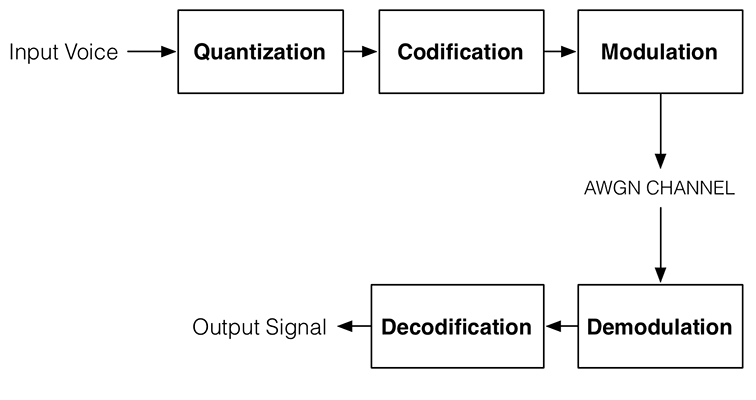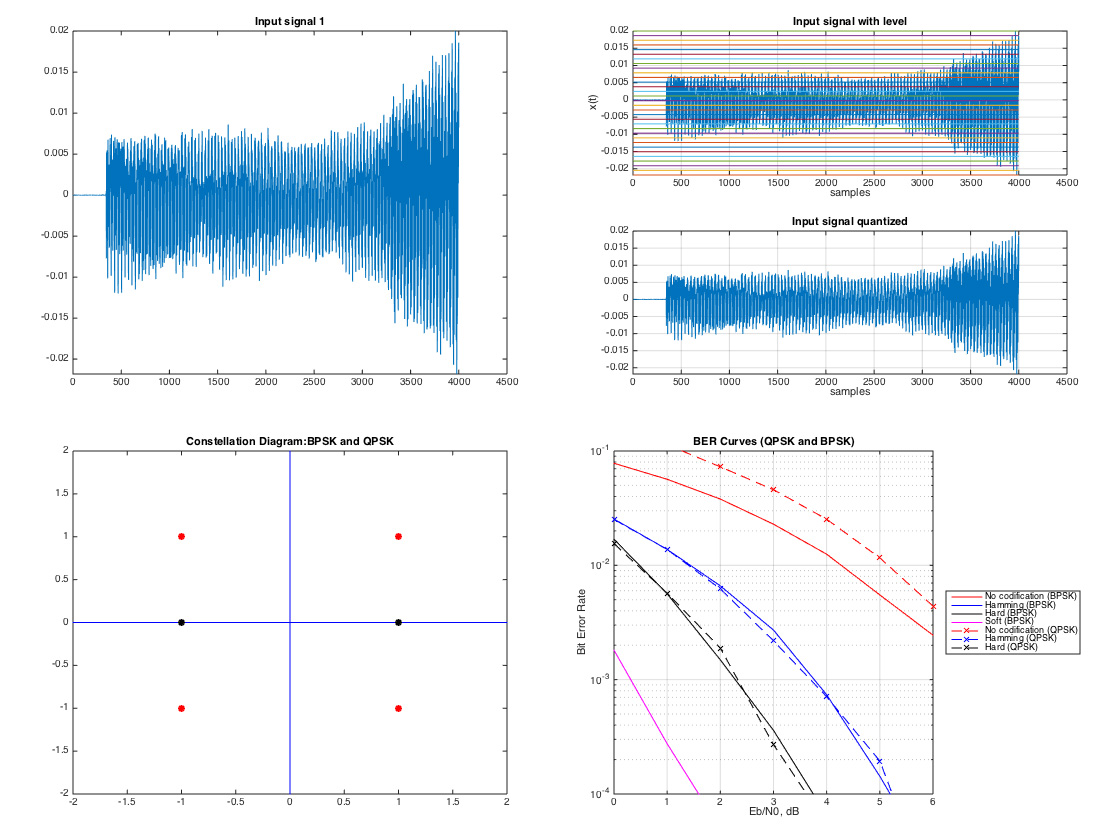This program simulates a basic communication system using MATLAB, and it plots BER curves in order to compare the performance of several codification algorithms. It includes the following components:This simulation not only enables you to visualize signals, but it also calculates performance metrics such as probability of error (Pe) and bit error rate (BER). Several algorithms are available for quantization, modulation and codification.

• Uniform
• Mu-Law
• A-Law

• BPSK
• QPSK

### Codification

• Hamming (7,4)
• Convolutional codes: soft decision
• Convolutional codes: hard decision

### GraphsIn case you’re interested, I also implemented a CDMA communication system.